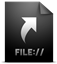9 years 8 years
27
Jan. 2013

### Boyer–Moore string search

Arkiv for denne måneden
###### Tags:
Python

In Digital Forensics 2 - 1st lecture one of the topics was how to search for similar words, words that are close to what we want. I remembered episode 203 of Security Now dedicated to a particular algorithm for improving the speed of search for exact strings.

The idea was instead of starting comparing a string character by character from the beginning of the search term, one should start at the end. If the last character does not match, a jump can be performed depending on a look up table that is very easy to generate. In this illustration, the old way uses 16 comparisons to find the match, while Boyer-Moore uses 8.I decided to implement it in python. It's not very succinct, nor do I think it's particularly fast, but it's a good way to understand how the method works. Download:(test strings)
size 12.0 KiB
sha256: f49ca8396a...b5013d834e

```# -*- coding: utf-8 -*-

"""Usage: "python search.py"Give it a fileSearch for terms, quit with "!q""""import sys

def generate_lookup_table(length_pattern):	# How far we can jump if compared character in haystack matches any of the chars in the pattern	lookup_table = {}	a = length_pattern - 2	i = 1	while a >= 0:		char = pattern[a]		if not char in lookup_table:			lookup_table[char] = i		a -= 1		i += 1

return lookup_table

def search(haystack, length_haystack, pattern, length_pattern, offset=0):	# length_haystack and length_pattern are input parameters to avoid unnecessary function calls	# offset is to continue from where the last hit was found	match = 0

if ((length_pattern < 1) or (length_haystack < 1)):		return 0

pos_pattern = length_pattern - 1	if offset:		pos_haystack = offset + length_pattern	else:		pos_haystack = pos_pattern

def verify_match(pos_pattern, pos_haystack):		# compare backwards until a match was found or a mismatch happens		while(pos_pattern >= 0):			#print("pos_haystack: %s (%s), pos_pattern: %s (%s)") % (pos_haystack, haystack[pos_haystack], pos_pattern, pattern[pos_pattern])			if(pattern[pos_pattern] != haystack[pos_haystack]):				return 0

pos_pattern -= 1			pos_haystack -= 1

# If no return yet, a match was found		return pos_haystack + 1

def optimal_jump(pos_haystack):		key = haystack[pos_haystack]		if key in lookup_table:			pos_haystack += lookup_table[key]		else:			pos_haystack += length_pattern

return pos_haystack

while(pos_haystack < length_haystack):		# Loop through the haystack, comparing the last char of the pattern...		#print("pos_haystack: %s (%s)") % (pos_haystack, haystack[pos_haystack])		if(pattern[pos_pattern] == haystack[pos_haystack]):			ret = verify_match(pos_pattern - 1, pos_haystack - 1)			if not ret:				pos_haystack = optimal_jump(pos_haystack)			else:				return ret		else:			pos_haystack = optimal_jump(pos_haystack)

return match

print('What file do you want to search in?:')filename = raw_input()try:	with open(filename, mode='rb') as content:		haystack_orig = content.read()except IOError, e:	print e	sys.exit()

preview = 30  # how much before and after the hit is reportedprogram_loop = 1while(program_loop):	print('\n\n\n\What you want to find: ("!q" to quit)')	pattern = raw_input()	if pattern != "!q":

result = []		cont = 1		offset = 0		haystack = haystack_orig.lower()		pattern = pattern.lower()		length_pattern = len(pattern)		length_haystack = len(haystack)		lookup_table = generate_lookup_table(length_pattern)

print("Searching for \"%s\" \nUsing lookup table%s") % (pattern, lookup_table)

while cont:			ret = search(haystack, length_haystack, pattern, length_pattern, offset)			if ret:				result.append(ret)				offset = ret			else:				cont = 0

if(result):			print "Match(es) found at position(s) %s" % result			for i in result:				low = i - preview				if low < 0:					low = 0				high = i + length_pattern + preview				if high > length_haystack:					high = length_haystack				print "\"%s\"" % haystack_orig[low:high]		else:			print "No match found!"

else:		program_loop = 0

```

Snarveier
Flere fra teknologi